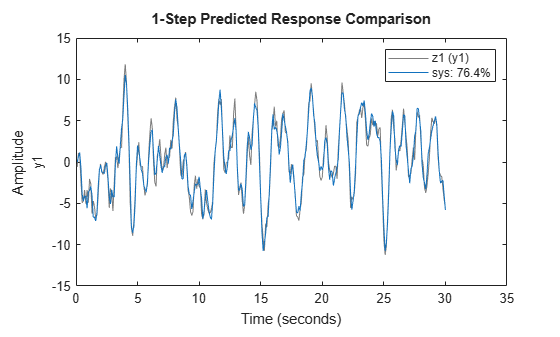Documentation

## Resolve Fit Value Differences Between Model Identification and `compare` Command

This example shows how NRMSE fit values computed by model identification functions and by the `compare` function can differ because of differences in initial conditions and prediction horizon settings.

When you identify a model, the model identification algorithm returns a value for the fit percentage to the measurement data you used. If you then use `compare` to plot the model simulation results against the measured data, fit results are not always identical. This difference is because:

• The model-identification algorithm and the `compare` algorithm use different methods to estimate initial conditions.

• The model-identification algorithm uses a default of one-step `prediction` focus, but `compare` uses a default of `Inf` — equivalent to a `simulation` focus. See Simulate and Predict Identified Model Output.

These differences are generally smalI, and they should not impact your model selection and validation workflow. If you do want to reproduce the estimation fit results precisely, you must reconcile the prediction horizon and initial condition settings used in `compare` with the values used during model estimation.

Load the data, and estimate a three-state state-space model.

```load iddata1 z1; sys = ssest(z1,3);```

Estimate a state-space model from the measured data.

Allow `ssest` to use its default `'Focus'` setting of `'prediction`', and its default `'InitialState'` estimation setting of 'auto'. The `'auto'` setting causes `ssest` to select:

• `'zero'` if the initial prediction error is not significantly higher than an estimation-based error, or if the system is FRD

• `'estimate'` for most single-experiment data sets, if `'zero'` is not acceptable

• `'backcast'` for multi-experiment data sets, if` 'zero'` is not acceptable

For more information, see `ssestOptions`.

You can retrieve the automatically selected initial-state option, the initial state vector, and the NRMSE fit percentage from the estimation report for `sys`.

`sys_is = sys.Report.InitialState`
```sys_is = 'zero' ```
`sys_x0 = sys.Report.Parameters.X0`
```sys_x0 = 3×1 0 0 0 ```
`sys_fit = sys.Report.Fit.FitPercent`
```sys_fit = 76.4001 ```

`ssest` selected `'zero'`, rather than estimating the initial states, indicating that the contribution of the initial states to prediction error is small.

Use `compare` to simulate the model and plot the results against the measured data. For this case, allow `compare` to use its own method for estimating initial conditions and its default of `Inf` for the prediction horizon.

```figure compare(z1,sys)```The NRMSE fit percentage computed by `compare` is lower than the fit that `ssest` computed. This lower value is primarily because of the mismatch between prediction horizons for `ssest` (default of `1`) and compare (default of `Inf`).

Rerun `compare` using an argument of `1` for the prediction horizon.

```figure compare(z1,sys,1)```The change to the prediction horizon resolved most of the discrepancy between fit values. Now the `compare` fit exceeds the `ssest` fit. This higher value is because `ssest` set initial states to 0, while `compare` estimated initial states. You can investigate how far apart the initial-state vectors are by rerunning `compare` with output arguments.

```[y1,fit1,x0c] = compare(z1,sys,1); x0c```
```x0c = 3×1 -0.0063 -0.0012 0.0180 ```

`x0c` is small, but not zero like `sys_x0`. To reproduce the `ssest` initial conditions in `compare`, directly set the `'InitialCondition'` option to `sys_x0`. Then run `compare` using the updated option set.

```compareOpt = compareOptions('InitialCondition',sys_x0); figure compare(z1,sys,1,compareOpt)```The fit result now matches the fit result from the original estimation.

This method confirms that the `ssest `algorithm and the `compare` algorithm yield the same results with the same initial-condition and prediction-horizon settings.

This example deals only with reproducing results when you want to confirm agreement between the estimation algorithm and the compare algorithm NRMSE fit values as they relate to the original estimation data. However, when you use `compare` to validate your model against validation data, or to compare the goodness of multiple candidate models, use the `compare `defaults for `'InitialCondition'` and prediction horizon as a general rule.